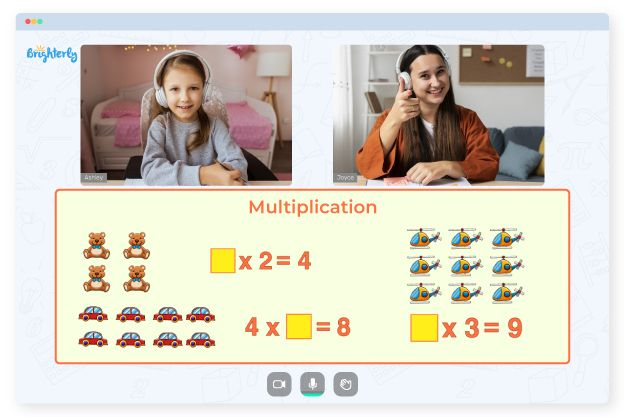# Multiplication and Division Worksheets Grade 3

The multiplication and division worksheets grade 3 is designed for third-grade students to practice and reinforce their understanding of multiplication and division concepts. Our 3rd grade multiplication and division worksheets include a variety of exercises and problems that require students to solve multiplication and division problems, both in isolation and in real-world contexts.

## What Is Multiplication and Division Worksheets for Grade 3?

The 3rd grade math worksheets multiplication and division include basic multiplication facts like 2 x 3 or 4 x 5 as well as more complex problems involving multi-digit multiplication like 23 x 46 or 567 x 28. The 3rd grade multiplication and division worksheets pdf also include word problems that require students to apply their multiplication skills to real-world situations.

Math for Kids

Is Your Child Struggling With Math?
1:1 Online Math TutoringAdditionally, our grade 3 multiplication and division worksheets cover basic division concepts, like dividing by 2, 5, and 10, as well as more advanced problems involving long division.

The mixed multiplication and division worksheets grade 3 are an important tool for helping students develop fluency and mastery in these essential mathematical operations. You can download them below to help your child practice at home.### Multiplication and Division Worksheets Grade 3 PDF

3rd Grade Math Worksheets Multiplication And Division### Multiplication and Division Worksheets Grade 3 PDF

3rd Grade Multiplication And Division Worksheets PDF### Multiplication and Division Worksheets Grade 3 PDF

3rd Grade Multiplication And Division Worksheets### Multiplication and Division Worksheets Grade 3 PDF

Grade 3 Multiplication And Division Worksheets

Struggling with Multiplication?• Is your child finding it difficult to understand multiplication?
• Consider lessons with an online tutor.

Does your child struggle with mastering multiplication? Start learning with an online tutor.